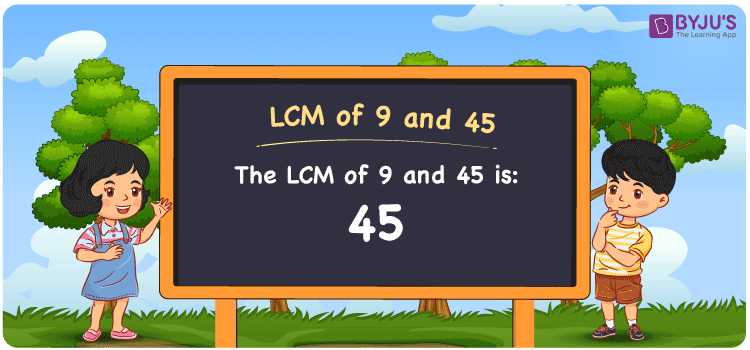Checkout JEE MAINS 2022 Question Paper Analysis : Checkout JEE MAINS 2022 Question Paper Analysis :

# LCM of 9 and 45

LCM of 9 and 45 is 45. Students can learn how to obtain the least common multiple of 9 and 45 from the common multiples. (9, 18, 27, 36, 45, 54, ….) and (45, 90, 135, 180,….) are the multiples of 19 and 45. The process which can be used to find the LCM of two numbers is prime factorisation, division and by listing the multiples.

## What is LCM of 9 and 45?

The answer to this question is 45. The LCM of 9 and 45 using various methods is shown in this article for your reference. The LCM of two non-zero integers, 9 and 45, is the smallest positive integer 45 which is divisible by both 9 and 45 with no remainder.## How to Find LCM of 9 and 45?

LCM of 9 and 45 can be found using three methods:

• Prime Factorisation
• Division method
• Listing the multiples

### LCM of 9 and 45 Using Prime Factorisation Method

The prime factorisation of 9 and 45, respectively, is given by:

9 = 3 x 3 = 3²

45 = 3 x 3 x 5 = 3² x 5¹

LCM (9, 45) = 45

### LCM of 9 and 45 Using Division Method

We’ll divide the numbers (9, 45) by their prime factors to get the LCM of 9 and 45 using the division method (preferably common). The LCM of 9 and 45 is calculated by multiplying these divisors.

 3 9 45 3 3 15 5 1 5 x 1 1

No further division can be done.

Hence, LCM (9, 45) = 45

### LCM of 9 and 45 Using Listing the Multiples

To calculate the LCM of 9 and 45 by listing out the common multiples, list the multiples as shown below:

 Multiples of 9 Multiples of 45 9 45 18 90 27 135 36 180 45 225 54 270

LCM (9, 45) = 45

## Video Lesson on Applications of LCM## LCM of 9 and 45 Solved Examples

Question: What is the smallest number divisible by 9 and 45 exactly?

Solution:

We know that

The smallest number exactly divisible by 9 and 45 is the LCM.

Multiples of 9 = 9, 18, 27, 36, 45, 54,…..

Multiples of 45 = 45, 90, 135, 180, ….

Hence, the LCM of 9 and 45 is 45.

## Frequently Asked Questions on LCM of 9 and 45

### In the numbers 45, 30, 28, 18, what is the LCM of 9 and 45?

The LCM value is the smallest common multiple which is evenly divisible by 9 and 45. The number which satisfies this condition is 45.

### Find the GCF if the LCM of 9 and 45 is 45.

LCM x GCF = 9 x 45

Given

LCM of 9 and 45 = 45

45 x GCF = 405

GCF = 405/45 = 9

### What is the relation between GCF and LCM of 9 and 45?

The relation between GCF and LCM of 9 and 45 is

LCM x GCF = 9 x 45

LCM x GCF = 405